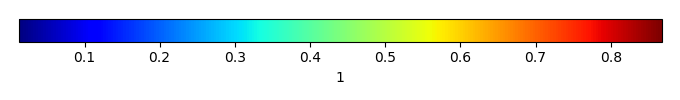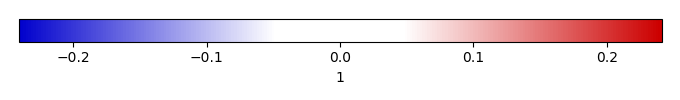# Mean State

Period Mean (original grids) 
Model Period Mean (intersection) 
Model Period Mean (complement) 
Benchmark Period Mean (intersection) 
Benchmark Period Mean (complement) 
Bias 
Phase Shift [months]
Bias Score 
Seasonal Cycle Score 
Spatial Distribution Score 
Overall Score 
Benchmark [-] 0.585
CLM5PHSOFF [-] 0.593 0.603 0.509 0.585 0.538 0.0171 1.94 0.464 0.681 0.992 0.712
CLM5PHSON [-] 0.583 0.593 0.496 0.585 0.538 0.00689 2.15 0.538 0.642 0.996 0.725
Period Mean (original grids) 
Model Period Mean (intersection) 
Model Period Mean (complement) 
Benchmark Period Mean (intersection) 
Benchmark Period Mean (complement) 
Bias 
Phase Shift [months]
Bias Score 
Seasonal Cycle Score 
Spatial Distribution Score 
Overall Score 
Benchmark [-] 0.425
CLM5PHSOFF [-] 0.465 0.469 0.221 0.424 0.597 0.0448 1.16 0.724 0.817 0.928 0.823
CLM5PHSON [-] 0.459 0.462 0.233 0.424 0.597 0.0383 1.32 0.710 0.780 0.918 0.803
Period Mean (original grids) 
Model Period Mean (intersection) 
Model Period Mean (complement) 
Benchmark Period Mean (intersection) 
Benchmark Period Mean (complement) 
Bias 
Phase Shift [months]
Bias Score 
Seasonal Cycle Score 
Spatial Distribution Score 
Overall Score 
Benchmark [-] 0.480
CLM5PHSOFF [-] 0.443 0.450 0.387 0.479 0.545 -0.0301 1.35 0.613 0.803 0.993 0.803
CLM5PHSON [-] 0.445 0.451 0.390 0.479 0.545 -0.0285 1.49 0.565 0.771 0.989 0.775
Period Mean (original grids) 
Model Period Mean (intersection) 
Model Period Mean (complement) 
Benchmark Period Mean (intersection) 
Benchmark Period Mean (complement) 
Bias 
Phase Shift [months]
Bias Score 
Seasonal Cycle Score 
Spatial Distribution Score 
Overall Score 
Benchmark [-] 0.436
CLM5PHSOFF [-] 0.449 0.450 0.442 0.436 0.461 0.0138 1.17 0.651 0.844 0.624 0.706
CLM5PHSON [-] 0.502 0.506 0.471 0.436 0.461 0.0700 1.07 0.540 0.882 0.474 0.632
Period Mean (original grids) 
Model Period Mean (intersection) 
Model Period Mean (complement) 
Benchmark Period Mean (intersection) 
Benchmark Period Mean (complement) 
Bias 
Phase Shift [months]
Bias Score 
Seasonal Cycle Score 
Spatial Distribution Score 
Overall Score 
Benchmark [-] 0.776
CLM5PHSOFF [-] 0.783 0.800 0.754 0.777 0.755 0.0223 2.23 0.265 0.649 0.541 0.485
CLM5PHSON [-] 0.732 0.744 0.711 0.777 0.755 -0.0326 1.87 0.296 0.717 0.691 0.568
Period Mean (original grids) 
Model Period Mean (intersection) 
Model Period Mean (complement) 
Benchmark Period Mean (intersection) 
Benchmark Period Mean (complement) 
Bias 
Phase Shift [months]
Bias Score 
Seasonal Cycle Score 
Spatial Distribution Score 
Overall Score 
Benchmark [-] 0.490
CLM5PHSOFF [-] 0.507 0.508 0.430 0.490 0.513 0.0178 1.19 0.674 0.834 0.994 0.834
CLM5PHSON [-] 0.551 0.552 0.460 0.490 0.513 0.0615 0.966 0.617 0.896 0.864 0.792
Period Mean (original grids) 
Model Period Mean (intersection) 
Model Period Mean (complement) 
Benchmark Period Mean (intersection) 
Benchmark Period Mean (complement) 
Bias 
Phase Shift [months]
Bias Score 
Seasonal Cycle Score 
Spatial Distribution Score 
Overall Score 
Benchmark [-] 0.463
CLM5PHSOFF [-] 0.384 0.411 0.250 0.463 0.376 -0.0524 1.82 0.500 0.717 0.991 0.736
CLM5PHSON [-] 0.420 0.450 0.263 0.463 0.376 -0.0130 1.75 0.563 0.726 0.971 0.753
Period Mean (original grids) 
Model Period Mean (intersection) 
Model Period Mean (complement) 
Benchmark Period Mean (intersection) 
Benchmark Period Mean (complement) 
Bias 
Phase Shift [months]
Bias Score 
Seasonal Cycle Score 
Spatial Distribution Score 
Overall Score 
Benchmark [-] 0.567
CLM5PHSOFF [-] 0.560 0.563 0.534 0.566 0.709 -0.00391 1.68 0.561 0.740 0.978 0.759
CLM5PHSON [-] 0.590 0.597 0.541 0.566 0.709 0.0303 2.07 0.541 0.664 0.983 0.729
Period Mean (original grids) 
Model Period Mean (intersection) 
Model Period Mean (complement) 
Benchmark Period Mean (intersection) 
Benchmark Period Mean (complement) 
Bias 
Phase Shift [months]
Bias Score 
Seasonal Cycle Score 
Spatial Distribution Score 
Overall Score 
Benchmark [-] 0.474
CLM5PHSOFF [-] 0.624 0.628 0.566 0.474 0.462 0.153 1.24 0.387 0.824 0.381 0.530
CLM5PHSON [-] 0.691 0.698 0.602 0.474 0.461 0.223 1.29 0.238 0.842 0.385 0.488
Period Mean (original grids) 
Model Period Mean (intersection) 
Model Period Mean (complement) 
Benchmark Period Mean (intersection) 
Benchmark Period Mean (complement) 
Bias 
Phase Shift [months]
Bias Score 
Seasonal Cycle Score 
Spatial Distribution Score 
Overall Score 
Benchmark [-] 0.317
CLM5PHSOFF [-] 0.341 0.327 0.439 0.316 0.553 0.0114 1.22 0.571 0.816 0.961 0.783
CLM5PHSON [-] 0.358 0.339 0.487 0.316 0.553 0.0232 1.26 0.607 0.803 0.987 0.799
Period Mean (original grids) 
Model Period Mean (intersection) 
Model Period Mean (complement) 
Benchmark Period Mean (intersection) 
Benchmark Period Mean (complement) 
Bias 
Phase Shift [months]
Bias Score 
Seasonal Cycle Score 
Spatial Distribution Score 
Overall Score 
Benchmark [-] 0.584
CLM5PHSOFF [-] 0.482 0.501 0.384 0.585 0.569 -0.0836 1.90 0.364 0.681 0.988 0.678
CLM5PHSON [-] 0.569 0.591 0.453 0.584 0.573 0.00699 2.03 0.513 0.654 0.993 0.720
Period Mean (original grids) 
Model Period Mean (intersection) 
Model Period Mean (complement) 
Benchmark Period Mean (intersection) 
Benchmark Period Mean (complement) 
Bias 
Phase Shift [months]
Bias Score 
Seasonal Cycle Score 
Spatial Distribution Score 
Overall Score 
Benchmark [-] 0.496
CLM5PHSOFF [-] 0.456 0.497 0.308 0.495 0.592 0.00157 1.71 0.500 0.728 0.984 0.737
CLM5PHSON [-] 0.478 0.523 0.314 0.495 0.590 0.0272 1.71 0.511 0.730 0.983 0.741
Period Mean (original grids) 
Model Period Mean (intersection) 
Model Period Mean (complement) 
Benchmark Period Mean (intersection) 
Benchmark Period Mean (complement) 
Bias 
Phase Shift [months]
Bias Score 
Seasonal Cycle Score 
Spatial Distribution Score 
Overall Score 
Benchmark [-] 0.251
CLM5PHSOFF [-] 0.129 0.259 0.0856 0.251 0.258 0.00778 2.83 0.447 0.495 0.924 0.622
CLM5PHSON [-] 0.143 0.306 0.0877 0.251 0.258 0.0542 3.03 0.398 0.438 0.964 0.600
Period Mean (original grids) 
Model Period Mean (intersection) 
Model Period Mean (complement) 
Benchmark Period Mean (intersection) 
Benchmark Period Mean (complement) 
Bias 
Phase Shift [months]
Bias Score 
Seasonal Cycle Score 
Spatial Distribution Score 
Overall Score 
Benchmark [-] 0.402
CLM5PHSOFF [-] 0.525 0.538 0.470 0.402 0.420 0.135 1.68 0.400 0.759 0.789 0.649
CLM5PHSON [-] 0.545 0.560 0.477 0.402 0.417 0.158 1.54 0.331 0.796 0.746 0.624
Period Mean (original grids) 
Model Period Mean (intersection) 
Model Period Mean (complement) 
Benchmark Period Mean (intersection) 
Benchmark Period Mean (complement) 
Bias 
Phase Shift [months]
Bias Score 
Seasonal Cycle Score 
Spatial Distribution Score 
Overall Score 
Benchmark [-] 0.505
CLM5PHSOFF [-] 0.517 0.510 0.532 0.505 0.531 0.00450 1.45 0.470 0.762 0.988 0.740
CLM5PHSON [-] 0.520 0.516 0.529 0.505 0.531 0.0102 1.54 0.508 0.732 0.983 0.741
Period Mean (original grids) 
Model Period Mean (intersection) 
Model Period Mean (complement) 
Benchmark Period Mean (intersection) 
Benchmark Period Mean (complement) 
Bias 
Phase Shift [months]
Bias Score 
Seasonal Cycle Score 
Spatial Distribution Score 
Overall Score 
Benchmark [-] 0.631
CLM5PHSOFF [-] 0.571 0.569 0.597 0.631 0.608 -0.0619 1.43 0.579 0.824 0.940 0.781
CLM5PHSON [-] 0.625 0.627 0.622 0.631 0.608 -0.00369 1.49 0.674 0.813 0.975 0.821
Period Mean (original grids) 
Model Period Mean (intersection) 
Model Period Mean (complement) 
Benchmark Period Mean (intersection) 
Benchmark Period Mean (complement) 
Bias 
Phase Shift [months]
Bias Score 
Seasonal Cycle Score 
Spatial Distribution Score 
Overall Score 
Benchmark [-] 0.473
CLM5PHSOFF [-] 0.497 0.500 0.469 0.472 0.514 0.0278 1.74 0.584 0.748 0.986 0.772
CLM5PHSON [-] 0.547 0.551 0.508 0.472 0.514 0.0793 1.28 0.524 0.841 0.986 0.784
Period Mean (original grids) 
Model Period Mean (intersection) 
Model Period Mean (complement) 
Benchmark Period Mean (intersection) 
Benchmark Period Mean (complement) 
Bias 
Phase Shift [months]
Bias Score 
Seasonal Cycle Score 
Spatial Distribution Score 
Overall Score 
Benchmark [-] 0.356
CLM5PHSOFF [-] 0.339 0.344 0.323 0.356 0.518 -0.0106 1.89 0.477 0.734 0.959 0.723
CLM5PHSON [-] 0.368 0.374 0.359 0.356 0.518 0.0187 1.73 0.489 0.755 0.985 0.743
Period Mean (original grids) 
Model Period Mean (intersection) 
Model Period Mean (complement) 
Benchmark Period Mean (intersection) 
Benchmark Period Mean (complement) 
Bias 
Phase Shift [months]
Bias Score 
Seasonal Cycle Score 
Spatial Distribution Score 
Overall Score 
Benchmark [-] 0.748
CLM5PHSOFF [-] 0.764 0.768 0.655 0.748 0.730 0.0192 2.77 0.311 0.548 0.670 0.509
CLM5PHSON [-] 0.744 0.749 0.626 0.748 0.679 4.92e-05 2.58 0.352 0.583 0.801 0.579
Period Mean (original grids) 
Model Period Mean (intersection) 
Model Period Mean (complement) 
Benchmark Period Mean (intersection) 
Benchmark Period Mean (complement) 
Bias 
Phase Shift [months]
Bias Score 
Seasonal Cycle Score 
Spatial Distribution Score 
Overall Score 
Benchmark [-] 0.631
CLM5PHSOFF [-] 0.586 0.584 0.588 0.631 0.630 -0.0458 1.96 0.518 0.686 0.836 0.680
CLM5PHSON [-] 0.603 0.600 0.618 0.631 0.630 -0.0304 2.21 0.560 0.639 0.850 0.683
Period Mean (original grids) 
Model Period Mean (intersection) 
Model Period Mean (complement) 
Benchmark Period Mean (intersection) 
Benchmark Period Mean (complement) 
Bias 
Phase Shift [months]
Bias Score 
Seasonal Cycle Score 
Spatial Distribution Score 
Overall Score 
Benchmark [-] 0.346
CLM5PHSOFF [-] 0.276 0.308 0.145 0.344 0.540 -0.0343 1.34 0.326 0.799 0.913 0.679
CLM5PHSON [-] 0.312 0.348 0.167 0.344 0.540 0.00626 1.28 0.321 0.809 0.883 0.671

# Temporally integrated period mean

BENCHMARK MEANMODEL MEANBIASBIAS SCOREBENCHMARK MAX MONTHMODEL MAX MONTHDIFFERENCE IN MAX MONTHSEASONAL CYCLE SCORESPATIAL TAYLOR DIAGRAMMODEL COLORS# Spatially integrated regional mean

MODEL COLORSREGIONAL MEANANNUAL CYCLEMONTHLY ANOMALYANNUAL CYCLE# All Models

BenchmarkCLM5PHSOFFCLM5PHSON# Data Information

Approach: I read variables latent heat (le) and sensible heat (sh) from monthly original data files from 1982 until 2008, then I calculated annual mean for both variables, and I further calculated evaporative fraction (le/(le+sh)) using annual mean for both le and sh I saved the data in NetCDF format.

Temporal resolution: climatology

General information: This product was derived originally from Monthly estimates of global biosphere-atmosphere fluxes from Biogeochemistry group at Max Planck Institute in Jena, Germany.

Spatial resolution: 0.5x0.5 degree